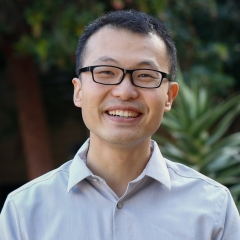Timezone: »

Optimal Uniform OPE and Model-based Offline Reinforcement Learning in Time-Homogeneous, Reward-Free and Task-Agnostic Settings
Ming Yin · Yu-Xiang Wang
This work studies the statistical limits of uniform convergence for offline policy evaluation (OPE) problems with model-based methods (for episodic MDP) and provides a unified framework towards optimal learning for several well-motivated offline tasks. We establish an $\Omega(H^2 S/d_m\epsilon^2)$ lower bound (over model-based family) for the global uniform OPE and our main result establishes an upper bound of $\tilde{O}(H^2/d_m\epsilon^2)$ for the \emph{local} uniform convergence. The highlight in achieving the optimal rate $\tilde{O}(H^2/d_m\epsilon^2)$ is our design of \emph{singleton absorbing MDP}, which is a new sharp analysis tool that works with the model-based approach. We generalize such a model-based framework to the new settings: offline task-agnostic and the offline reward-free with optimal complexity $\tilde{O}(H^2\log(K)/d_m\epsilon^2)$ ($K$ is the number of tasks) and $\tilde{O}(H^2S/d_m\epsilon^2)$ respectively. These results provide a unified solution for simultaneously solving different offline RL problems.

#### Author Information

##### Yu-Xiang Wang (UC Santa Barbara)Yu-Xiang Wang is the Eugene Aas Assistant Professor of Computer Science at UCSB. He runs the Statistical Machine Learning lab and co-founded the UCSB Center for Responsible Machine Learning. He is also visiting Amazon Web Services. Yu-Xiang’s research interests include statistical theory and methodology, differential privacy, reinforcement learning, online learning and deep learning.